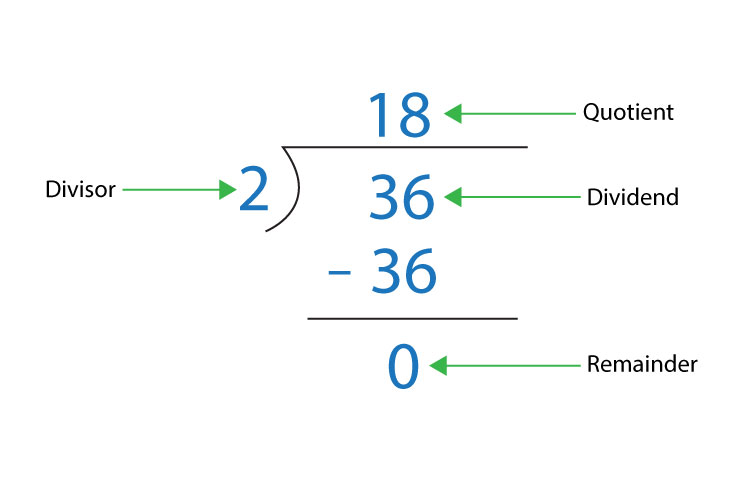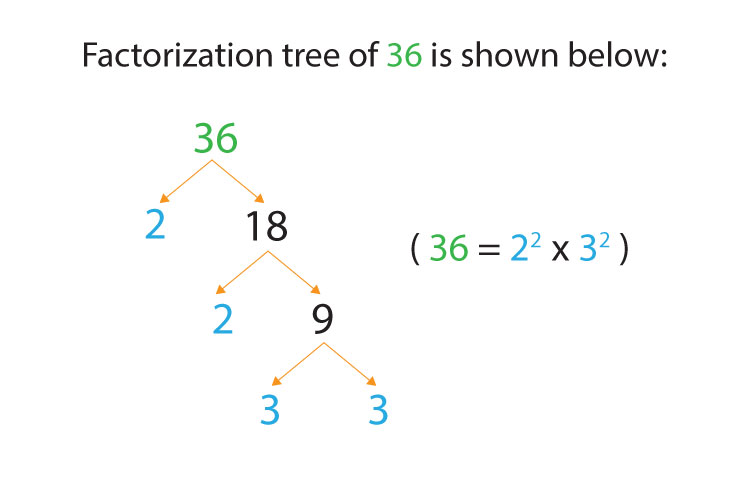# Write 36 as a product of prime factors. Give your answer in index form.

Prime factorization is a way of expressing a number as a product of its prime factors. A prime number is a number that has exactly two factors, 1 and the number itself. Factor is a number that divides another number evenly i.e., with no remainder.

Question:

A factor tree is a diagram used to determine the prime factors of a natural number greater than one.

1.   Divide 36 by 2

36 / 2 = 18quotient * divisor = dividend

18 * 2 = 36

2.   Write 36 as12 * 2 in the factor tree. 2 is a prime number so divide 18 by 2 = 9

3.   Write 12 as 2 * 9 in the factor tree. 2 is a prime number so divide 9 by 2. 9 cannot be evenly divided by 2 so divide 9 by 3 = 3.

4.   Write 6 as 3 * 3 in the factor tree. 3 and 3 are prime factors so it is fully factorized.5.   An index number is a number which is raised to a power.

e.g. m * m * m * m* m = m5

6.   36 = 2 * 2 * 3 * 3

36 = 22 * 32